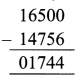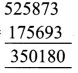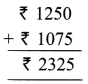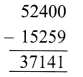# DAV Class 4 Maths Chapter 2 Worksheet 8 Solutions

The DAV Class 4 Maths Book Solutions and DAV Class 4 Maths Chapter 2 Worksheet 8 Solutions of Addition and Subtraction offer comprehensive answers to textbook questions.

## DAV Class 4 Maths Ch 2 WS 8 Solutions

Question 1.
Solve the following word problems.
(a) There are 48,570 plants in a nursery. Its adjoining nursery has 51,257 plants. Which nursery has more plants and by how much.
Total no. of plants in nursery = 48,570
Total no. of plants in adjoining nursery = 51,257
51,257 > 48,570
So, adjoining nursery has more plants.
No. of plants more in adjoining nursery =Thus, 2,687 plants are more in the adjoining nursery.

(b) In 2011, the population of a town was 5,75,890. If the number of males was 2,98,170, find the number of females in the town.
Total population of town in 2011 = 5 7 5 8 9 0
No. of males = 298 1 70
No. of females = 2 7 7 7 2 0Thus, the number of females in the town is 2,77,720

(c) How much more is 16,500 than 14,756?
1st number = 16500
2nd number = 14756
Difference = 01744Thus, 16,500 is 1,744 more than 14,756.

(d) What must be subtracted from 5,25,873 to get 1,75,693?
As 5,25,873 > 1,75,693
So 1st number = 525873
2nd number = 175693
Difference = 350180Thus, 3,50,180 must be subtracted from 5,25,873 to get 1,75,693.

### DAV Class 4 Maths Chapter 2 Value Based Questions

Question 1.
How much money did Amol and Deepak give to Krishna?
Money given by Amol = ₹ 1250
Money given by Deepak = ₹ 1075Hence, Amol and Deepak gave ₹ 2,325 to Krishna.

Question 2.
If Krishna needed ₹ 2,000 for his new books and uniform, how much money is left with him?
Money needed for new books and uniform = ₹ 2,000
Money left with Krishna = 2,325 – 2,000
= 325
Hence, money left with Krishna is ₹ 325.

Question 3.
How do you feel when you help others?
We feel very nice and satisfied when we help others.

###Word Problem

E.g.
There are 52,400 bags of cement in a godown. Out of these 15,259 bags were sent to market. How many bags of cement remained in godown?